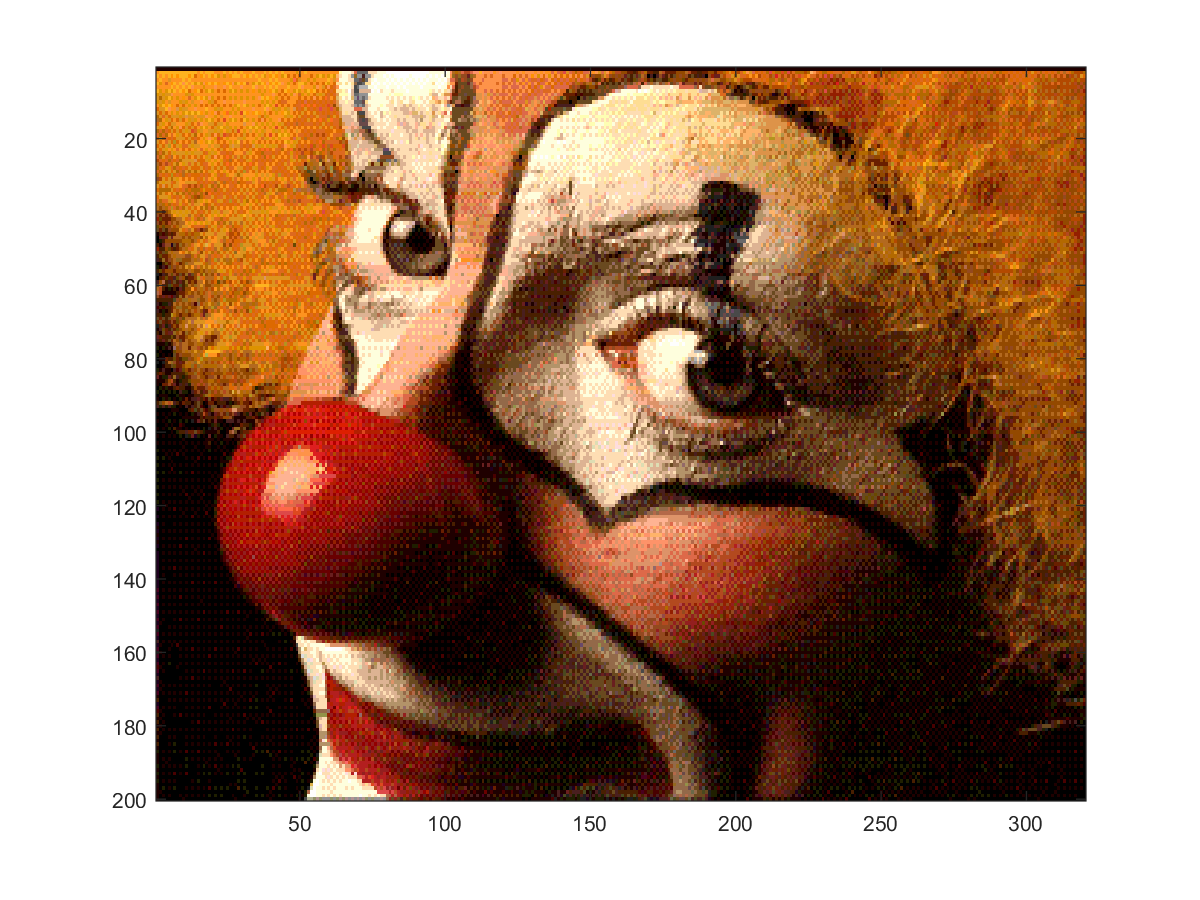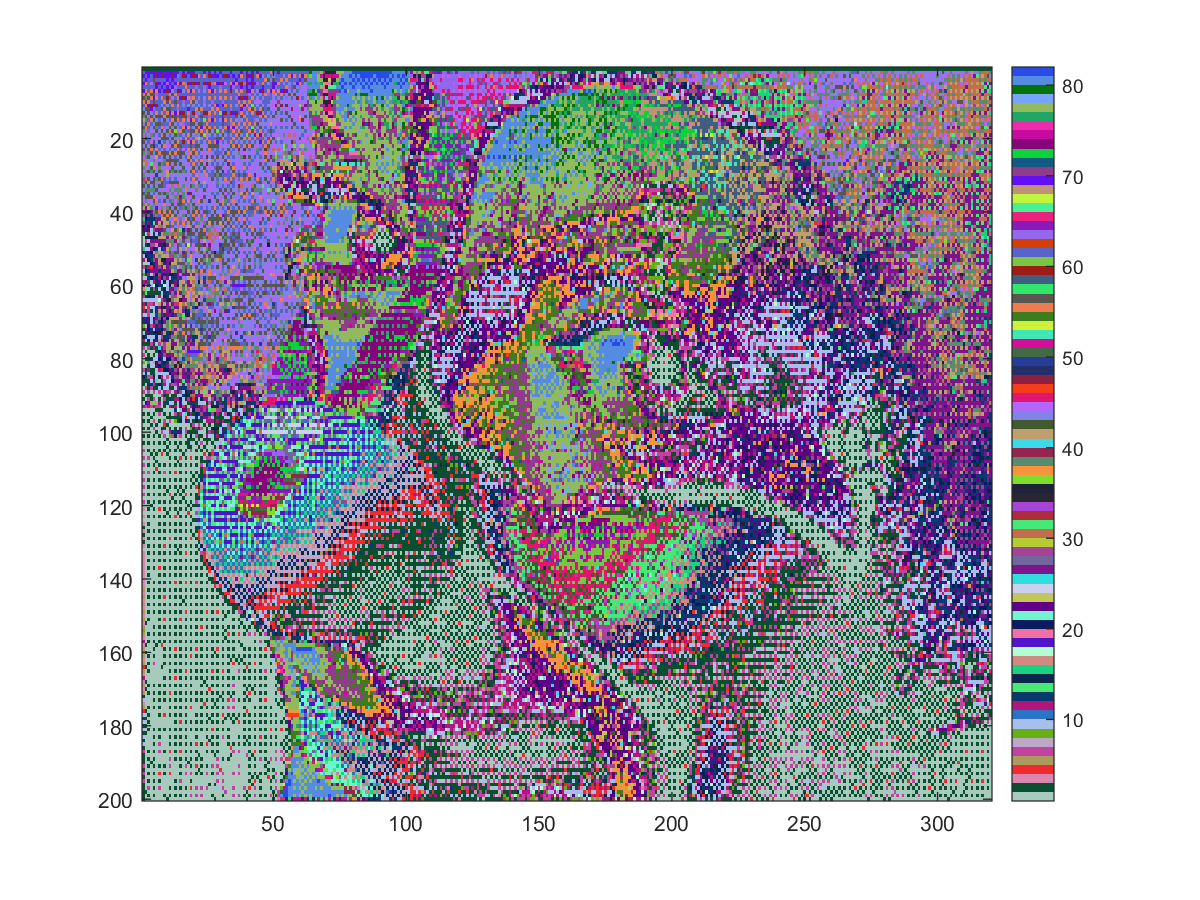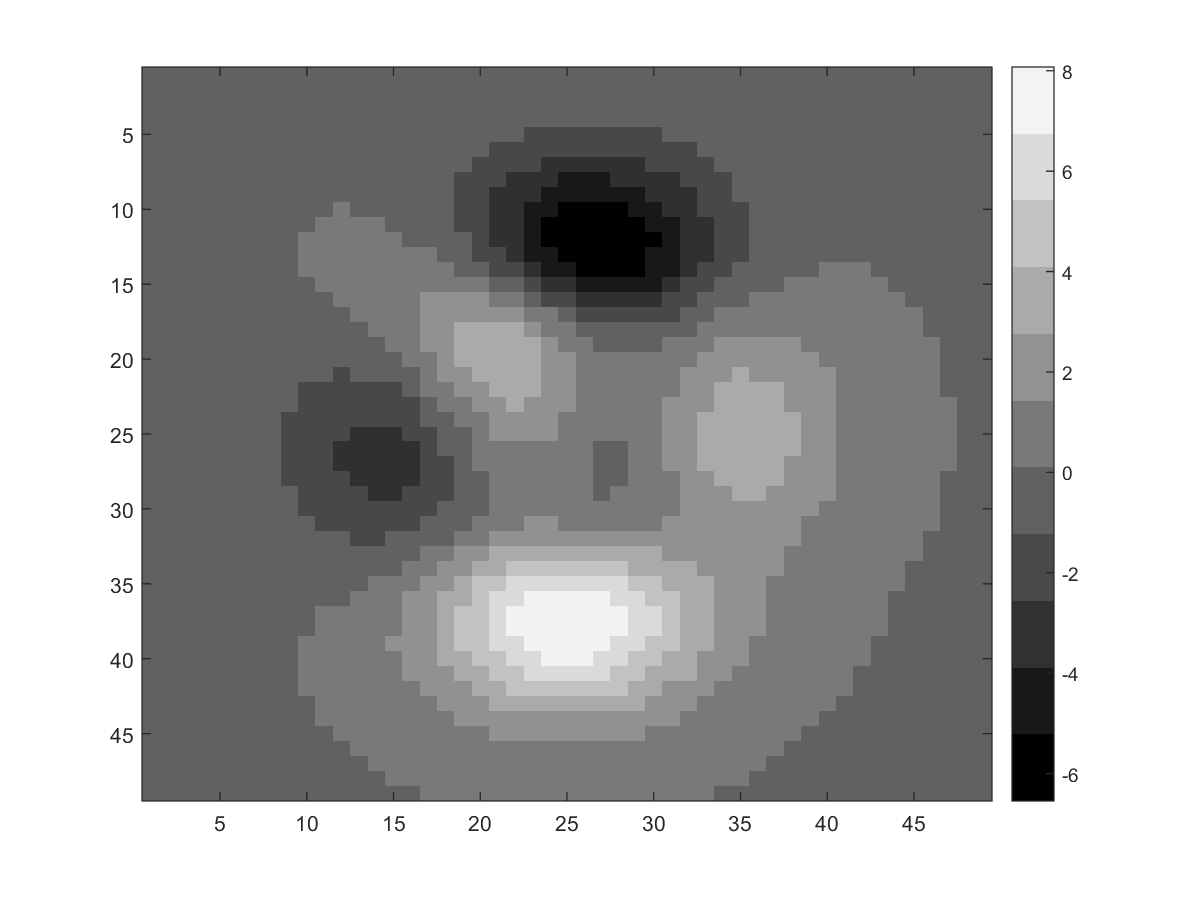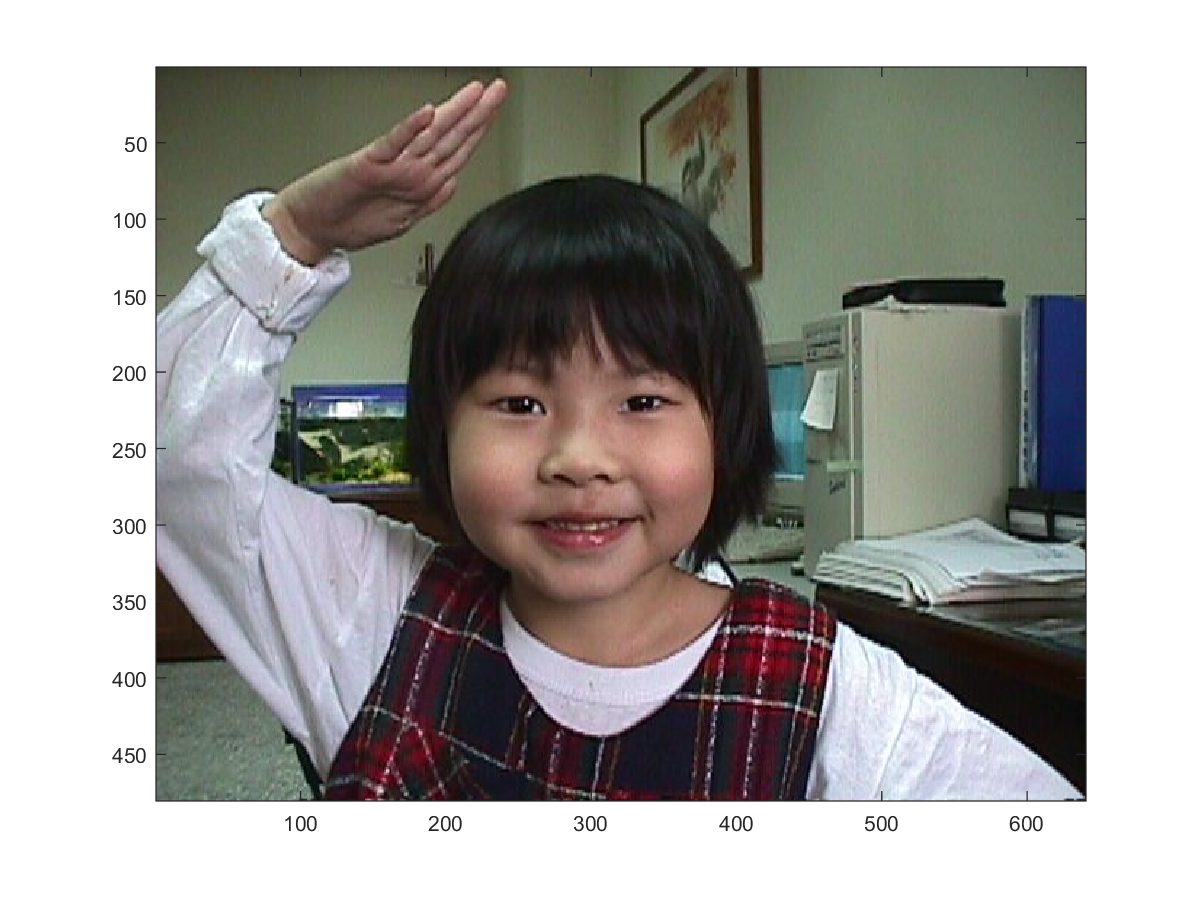19-1 MATLAB 嚙踝蕭嚙踝蕭嚙踝蕭嚙踝蕭嚙踝蕭嚙踝蕭

MATLAB 最常處理的影像格式為索引影像（Indexed Images），欲顯示此類影像時，使用語法如下：

image(X); colormap(map);

Hint
MATLAB 有現成的色盤矩陣（或顏色對照表），請參考本書第四章「三維立體繪圖」的第三節「曲面顏色的控制」。

Example 1: 19-影像顯示與讀寫/image01.mload clown.mat % 載入小丑影像資料，含變數 X 和 map image(X); % 顯示影像 colormap(map) % 取用色盤矩陣Example 2: 19-影像顯示與讀寫/image02.mload clown.mat % 載入小丑影像資料，含變數 X 和 map fprintf('min(min(X)) = %d\n', min(min(X))); fprintf('max(max(X)) = %d\n', max(max(X))); fprintf('size(map, 1) = %d\n', size(map, 1));min(min(X)) = 1 max(max(X)) = 81 size(map, 1) = 81

Example 3: 19-影像顯示與讀寫/image03.mload clown newmap = rand(size(map)); image(X); colormap(newmap); colorbar;Example 4: 19-影像顯示與讀寫/imagesc01.mtemp=colormap(gray); newMap=temp(1:6:end, :); X = peaks; colormap(newMap); imagesc(X); colorbar; min(min(X)) % 顯示 X 的最小值 max(max(X)) % 顯示 X 的最大值 ans = -6.5466 ans = 8.0752Hint
peaks 指令可以產生一個凹凸有緻的曲面，詳見本書第四章「三維立體繪圖」的說明。

Example 5: 19-影像顯示與讀寫/image04.m%X = imread('ngc6543a.jpg'); X = imread('annie19980405.jpg'); image(X) size(X) ans = 480 640 3Hint

MATLAB程式設計：入門篇